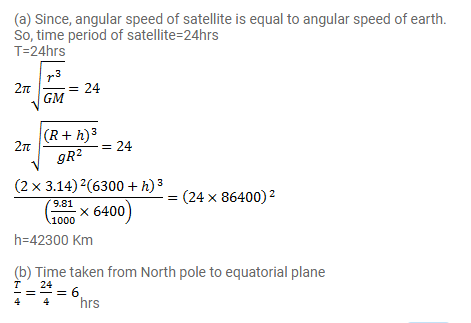# (a) Find the radius of the circular orbit of a satellite moving with

Question:

(a) Find the radius of the circular orbit of a satellite moving with an angular speed equal to the angular speed of earth's rotation.

(b) If the satellite is directly above the north pole at some instant, find the time it takes to come over the equatorial plane. Mass of the earth $=6 \times 10^{24} \mathrm{~kg}$.

Solution: